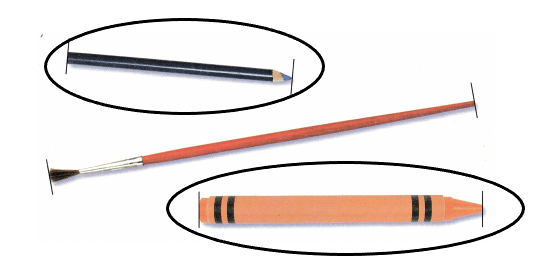Refer to our Texas Go Math Grade 2 Answer Key Pdf to score good marks in the exams. Test yourself by practicing the problems from Texas Go Math Grade 2 Unit 4 Assessment Answer Key.

Concepts and Skills

Question 1.
Circle shapes that have exactly 4 sides. Draw an X on shapes that have more than 4 vertices. (TEKS 2.8.C)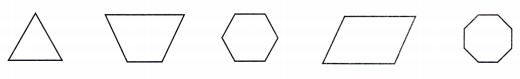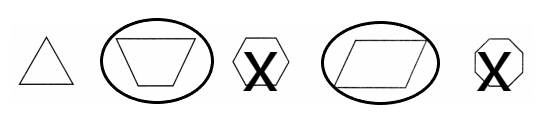Explanation:
I circled the shapes that have only 4 sides and marked X on the shapes that have more than 4 vertices.

Use a ruler to draw the shape. (TEKS 2.8.A)

Question 2.
a shape with 6 sides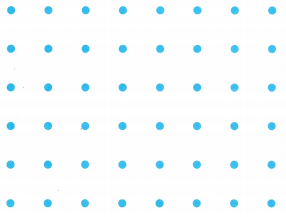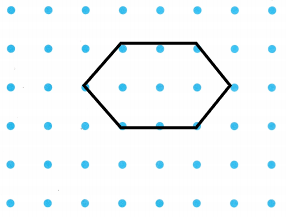Explanation:
I drew a hexagon that is a 6 sided shape.

Question 3.
a shape with 4 vertices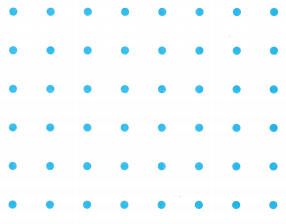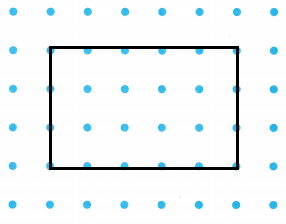Explanation:
I drew a rectangle. A rectangle has 4 vertices.

Question 4.
Build a rectangular prism with 24 cubes. Shade to show the top and front views. (TEKS 2.8.D)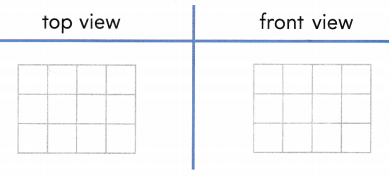Fill in the bubble for the correct answer choice.

Question 5.
Which shape shows a way to fold and cut a pentagon to make two quadrilaterals? (TEKS 2.8.E)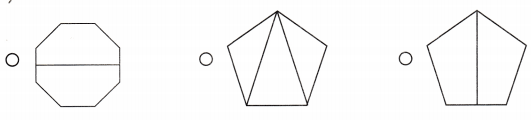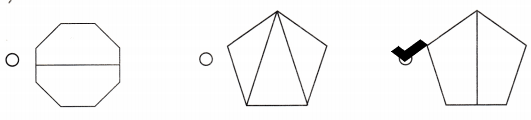Explanation:
I marked the shape that shows a way to fold and cut a pentagon to make two quadrilaterals.

Question 6.
Max starts baseball practice at the time shown on the clock. At what time did he start baseball practice? (TEKS 2.9.G)(A) 3:55
(B) 2:55
(C) 11:15
(B) 2:55

Explanation:
The above clock has the hours hand on 2 and the minutes hand on 11 which tells it is 55 minutes. So, the time shown in the clock is 2:55.

Question 7.
Which of these solids is a rectangular prism? (TEKS 2.8.B)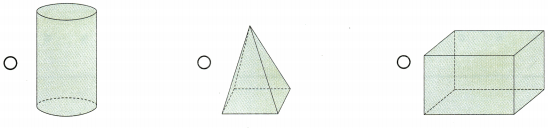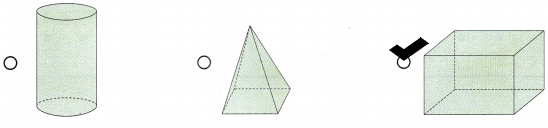Explanation:
I marked the  3rd shape as it is called  a rectangular prism.

Question 8.
Which of the following shapes has 8 sides? (TEKS 2.8.C)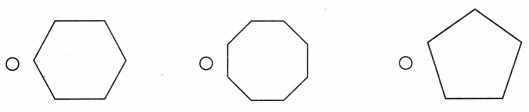Explanation:
I marked the shape that has 8 sides.

Question 9.
David measures a rope in centimeters. He draws this diagram to show the length of the rope. How many centimeters long is his rope? (TEKS 2.9.C)(A) 16 centimeters
(B) 21 centimeters
(C) 5 centimetersExplanation:
David measures a rope in centimeters. He draws this diagram to show the length of the rope
I counted the measurements by grouping them.
5+5+5+1=16
Therefore, David’s rope is 16 centimeters long.

Question 10.
Ellen is telling her sister about using a ruler. Which sentence is true? (TEKS 2.9.B)
(A) I foot is the same as I meter.
(B) I foot is the same as 12 inches.
(C) I centimeter is the same as 10 meters.
(B) I foot is the same as 12 inches.

Explanation:
An inch ruler is used to measure any object in inches and 12 inches makes 1 feet.

Question 11.
Use an inch ruler. What is the length of the yarn to the nearest inch? (TEKS 2.9.D)(A) 4 inches
(B) 40 inches
(C) 6 inches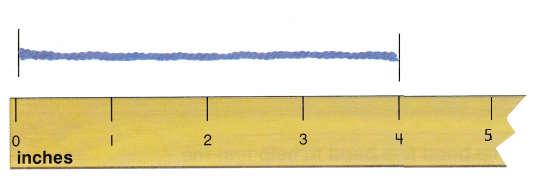Explanation:
I used an inch ruler and the yarn is 4 inches long.

Question 12.
Olivia brushes her teeth at this time each night. Which is this time shown on the clock? (TEKS 2.9.G)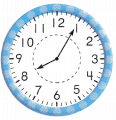(A) 1:40 p.m.
(B) 8:05 a.m.
(C) 8:05 p.m.
(C) 8:05 p.m.

Explanation:
Olivia brushes her teeth at this time each night
In the above clock the hours hand is on 8 and the minutes hand is on 1 which means 5 minutes.
The clock shows that it is 8:05 and as it is night the time is 8:05pm
Therefore, Olivia brushes her teeth at 8:05 pm.

Question 13.
Adrian is measuring some classroom objects. Use an inch ruler. Circle two objects below that have a total length that is less than 9 inches. (TEKS 2.9.D)